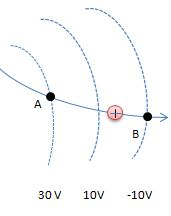# A proton's speed as it passes point A is 35000 m/s. It follows the trajectory shown in the...

## Question:

A proton's speed as it passes point A is 35000 m/s. It follows the trajectory shown in the figure.What is the proton's speed at point B?

## Electrostatic Potential Energy:

The energy of the charge in the electric field is known as the electrostatic potential energy. It is also given as the product of the charge and the difference of the electric potential.

Given Data:

• Speed of proton at A = 35000 m/s
• Mass of proton, {eq}m = 1.67 \times 10^{-27} {/eq}
• Potential at A = 30 V
• Potential at B = - 10 V
• Change in the potential {eq}\rm \delta V = V_{B} - V_{A} \\ \delta V = -10 - 30 \\ \delta V = -40 \ V {/eq}

Now, the decrease in the potential energy will lead to increase the kinetic energy if the proton

Therefore, applying the energy conservation

{eq}\rm \dfrac{1}{2}mu^{2} = \dfrac{1}{2}mv^{2} + U \\ \dfrac{1}{2}mv^{2} = \dfrac{1}{2}mu^{2} - U \\ \dfrac{1}{2}(m)(v^{2}) = \dfrac{1}{2}mu^{2} - (\delta V \times q ) \\ 0.5 \times (1.67 \times 10^{-27} \ kg)v^{2} = [ 0.5 \times (1.67 \times 10^{-27} ) \times 35000^{2} ] - [ (-40) \times (1.6 \times 10^{-19}) ] \\ v = 94285.05 \ m/s {/eq}